Ex 12.2

Chapter 12 Class 10 Areas related to Circles
Serial order wise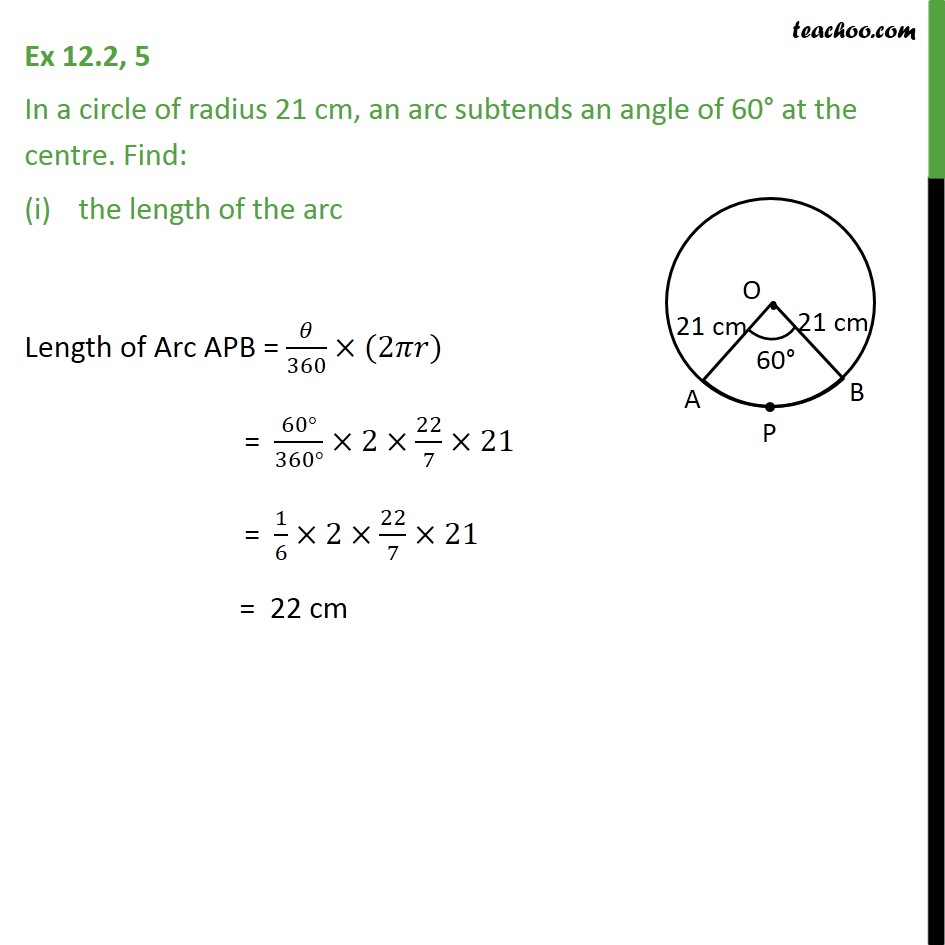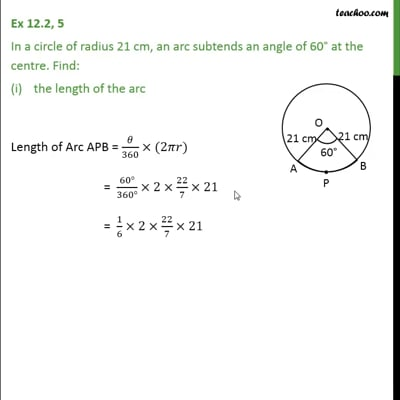This video is only available for Teachoo black users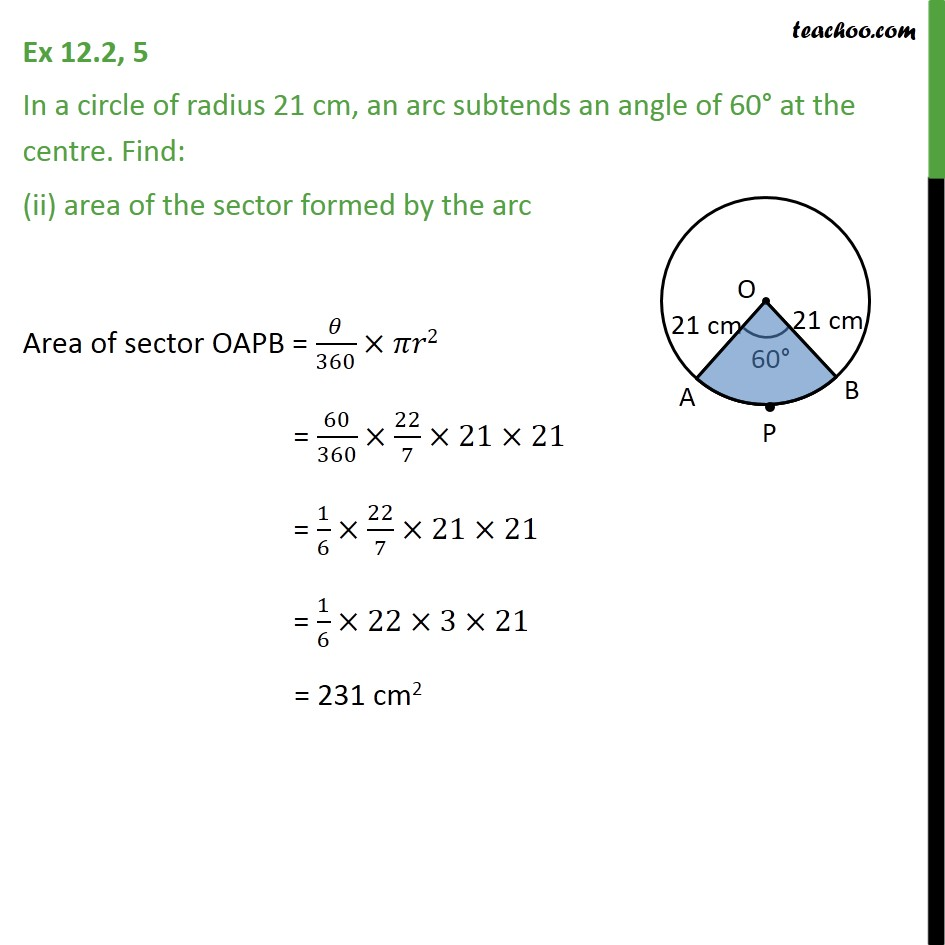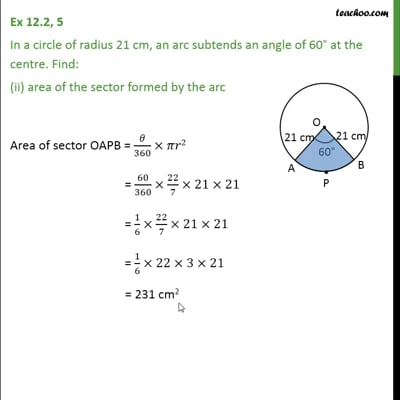This video is only available for Teachoo black users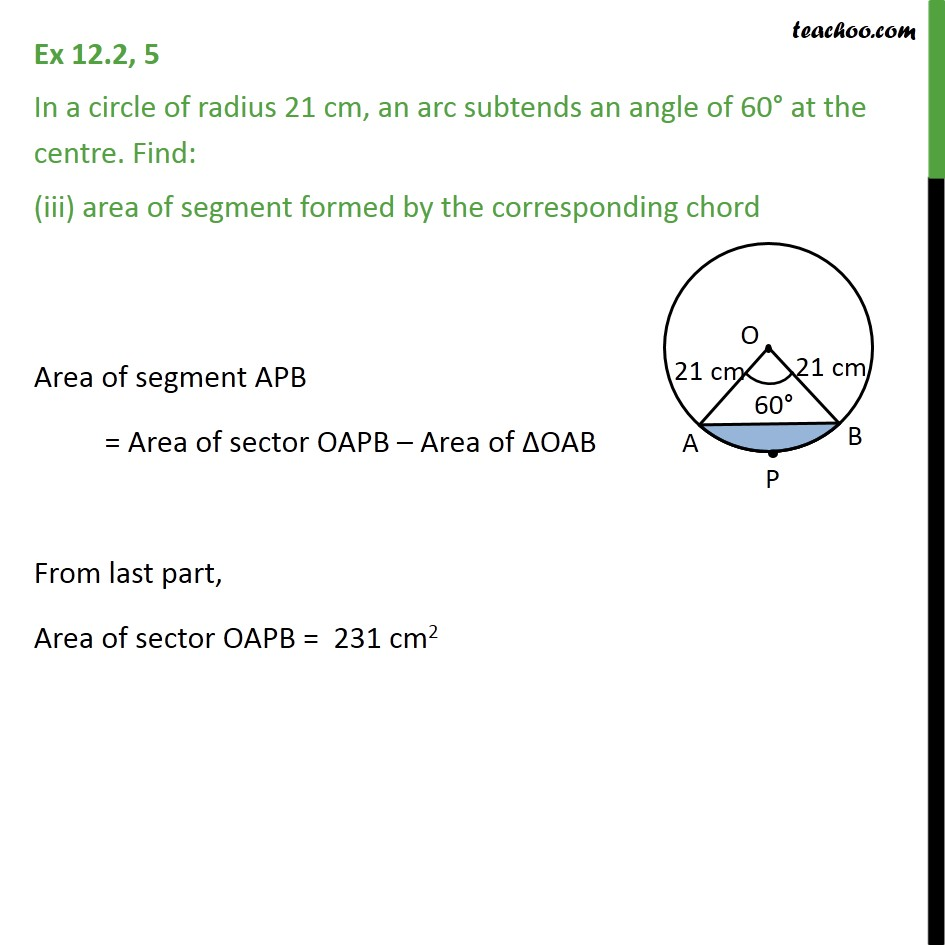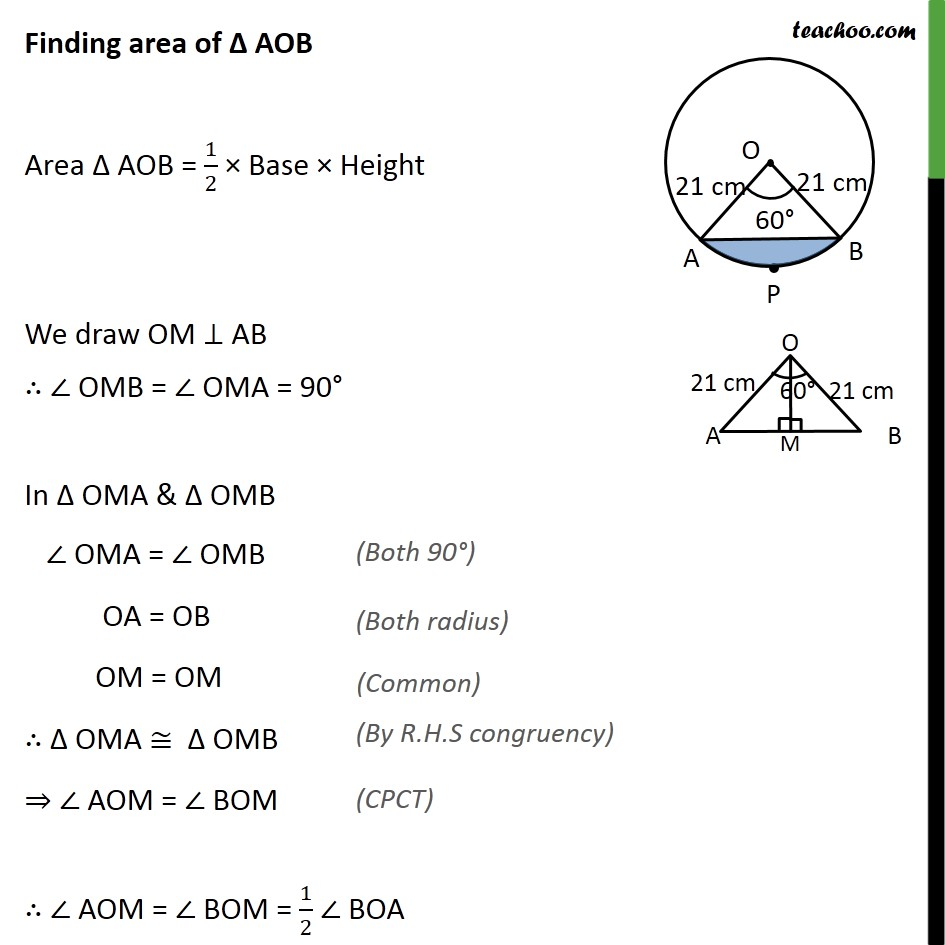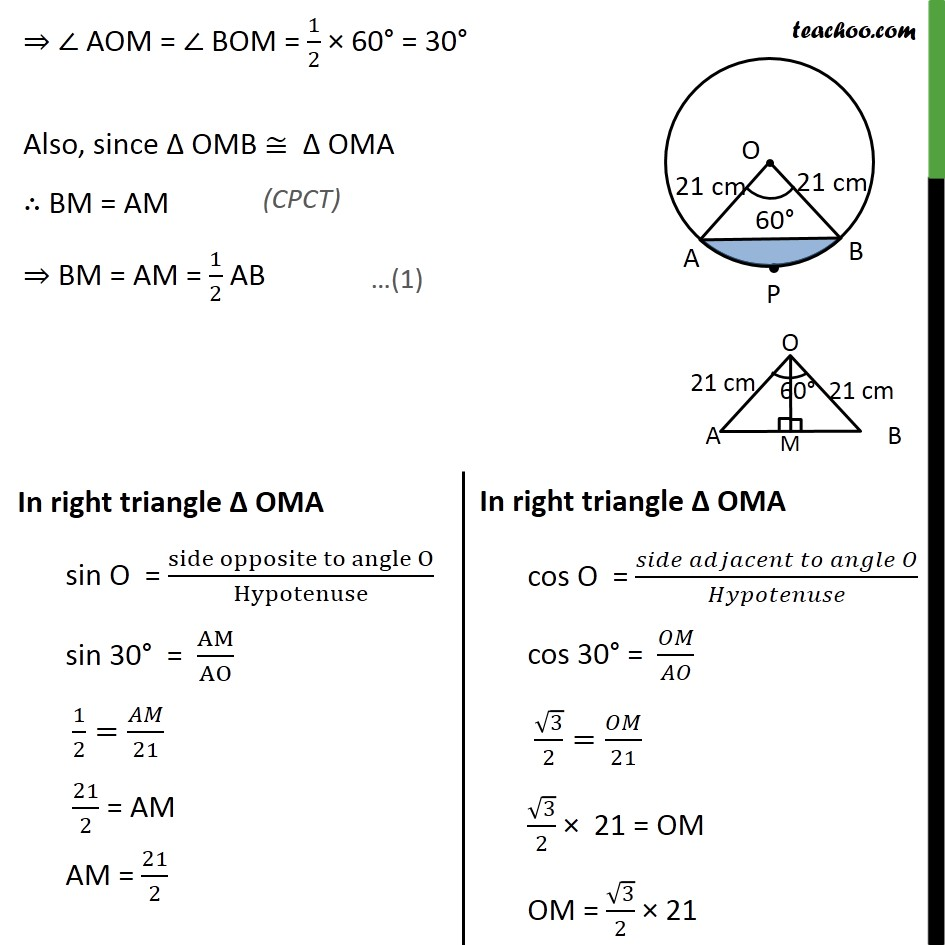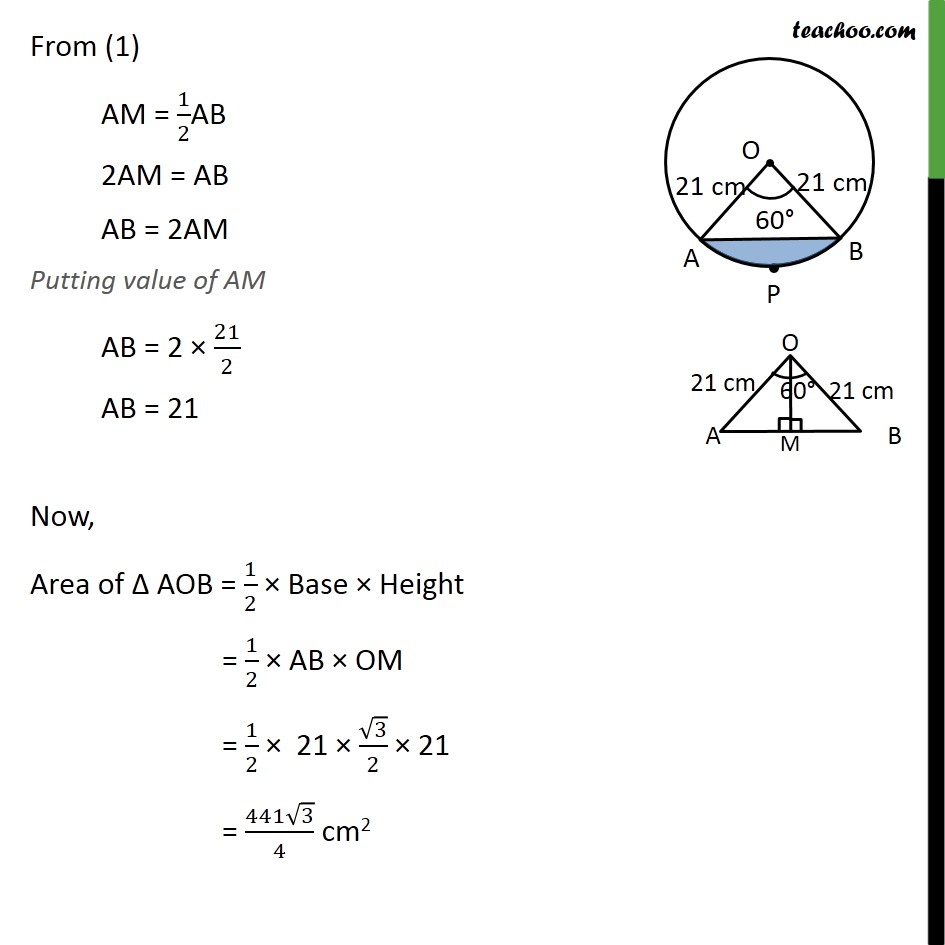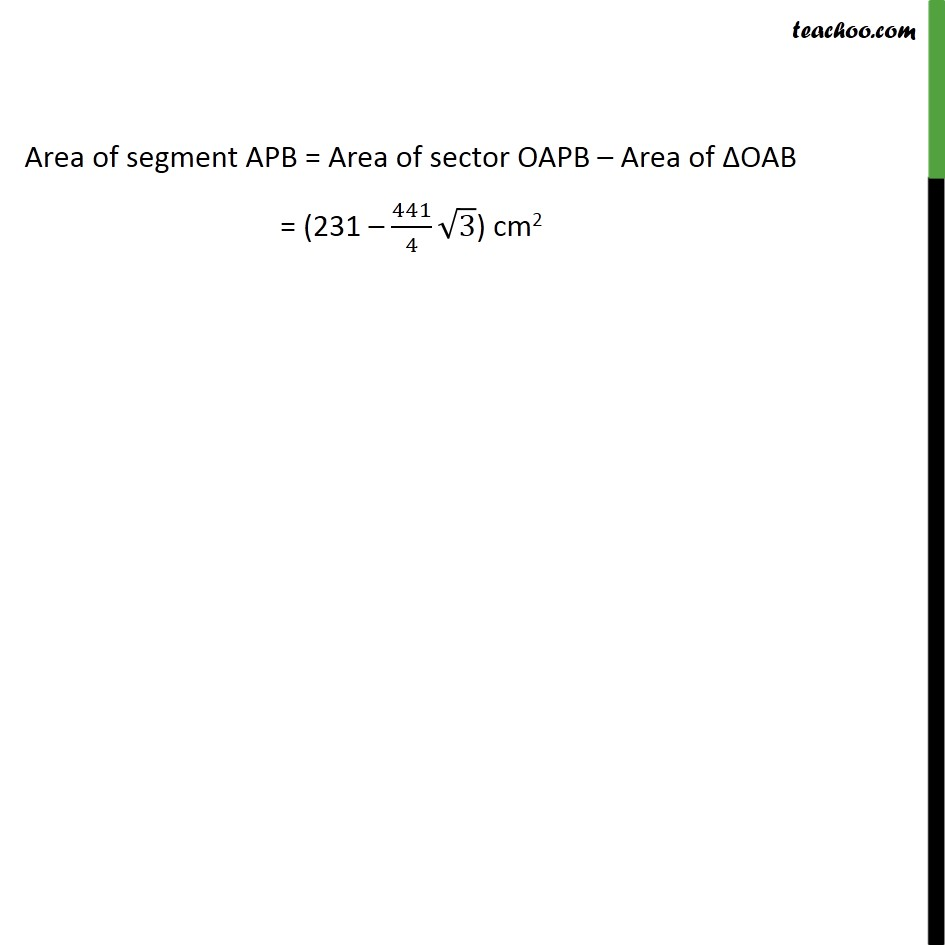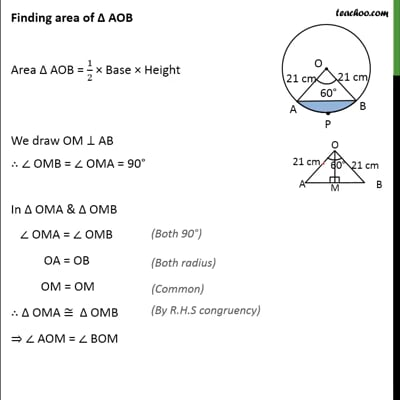This video is only available for Teachoo black users

Get live Maths 1-on-1 Classs - Class 6 to 12

### Transcript

Ex 12.2, 5 In a circle of radius 21 cm, an arc subtends an angle of 60 at the centre. Find: the length of the arc Length of Arc APB = /360 (2 ) = (60 )/(360 ) 2 22/7 21 = 1/6 2 22/7 21 = 22 cm Ex 12.2, 5 In a circle of radius 21 cm, an arc subtends an angle of 60 at the centre. Find: (ii) area of the sector formed by the arc Area of sector OAPB = /360 2 = 60/360 22/7 21 21 = 1/6 22/7 21 21 = 1/6 22 3 21 = 231 cm2 Ex 12.2, 5 In a circle of radius 21 cm, an arc subtends an angle of 60 at the centre. Find: (iii) area of segment formed by the corresponding chord Area of segment APB = Area of sector OAPB Area of OAB From last part, Area of sector OAPB = 231 cm2 Finding area of AOB Area AOB = 1/2 Base Height We draw OM AB OMB = OMA = 90 In OMA & OMB OMA = OMB OA = OB OM = OM OMA OMB AOM = BOM AOM = BOM = 1/2 BOA AOM = BOM = 1/2 60 = 30 Also, since OMB OMA BM = AM BM = AM = 1/2 AB From (1) AM = 1/2AB 2AM = AB AB = 2AM Putting value of AM AB = 2 21/2 AB = 21 Now, Area of AOB = 1/2 Base Height = 1/2 AB OM = 1/2 21 3/2 21 = (441 3)/4 cm2 Area of segment APB = Area of sector OAPB Area of OAB = (231 441/4 3) cm2